# RNAseq Visualization Automation

Install RVA from GitHub

devtools::install_github("THERMOSTATS/RVA_prod")

library(RVA)

Let’s load a summary statistics tables and combine them into a list named d1.

df <- RVA::Sample_summary_statistics_table
df1 <- RVA::Sample_summary_statistics_table1
d1 <- list(df, df1)

This is head of the first summary statictic table present in the list:

logFC AveExpr t P.Value adj.P.Val B
ENSG00000123610.5 -1.2886593 4.306067 -8.647905 0 0 28.14522
ENSG00000148926.10 -0.9519794 6.083623 -8.015885 0 0 23.54129
ENSG00000141664.9 -0.8942611 5.356978 -7.922250 0 0 22.86899
ENSG00000104320.13 -0.5723190 4.574599 -7.853658 0 0 22.36399
ENSG00000120217.14 -1.2170891 3.112864 -7.874408 0 0 22.31510
ENSG00000152778.9 -0.9307776 4.302267 -7.771144 0 0 21.76999

The row names are gene id, the supported gene id can be one of: ACCNUM, ALIAS, ENSEMBL, ENSEMBLPROT, ENSEMBLTRANS, ENTREZID, ENZYME, EVIDENCE, EVIDENCEALL, GENENAME, GO, GOALL, IPI, MAP, OMIM. For the provided sample datasets in this package we only have ENSEMBL id’s for gene id type.

# Functions

## Cutoff Plot

This function checks the number of differencialy expressed (DE) genes at different cutoff combinations. It process summary statistics table generated by differential expression analysis like limma or DESeq2, as input data, to evaluate the number of differntially expressed genes with different FDR and fold change cutoff.

Below are the default parameters for plot_cutoff. You can change them to modify your output. Use help(plot_cutoff) to learn more about the parameters.

plot_cutoff(data = data,
comp.names = NULL,
FCflag = "logFC",
FCmin = 1.2,
FCmax = 2,
FCstep = 0.1,
p.min = 0,
p.max = 0.2,
p.step = 0.01,
plot.save.to = NULL,
gen.3d.plot = TRUE,
gen.plot = TRUE)

### 1.1 Cutoff Plot - Input: a data frame.

cutoff.result <- plot_cutoff(data = df,
gen.plot = TRUE,
gen.3d.plot = TRUE)

The result object cutoff.result takes a data frame as an input data and contains 3 objects:

1. A table that summarizes the number of DE genes under threshold combination

head(cutoff.result[])
pvalue FC Number_of_Genes
0 1.2 0
0.01 1.2 1135
0.02 1.2 1246
0.03 1.2 1302
0.04 1.2 1345
0.05 1.2 1388

2. A 3D plotly object, where the x-axis is Fold change threshold, y-axis is FDR cutoff, and z-axis is the number of DE genes under the x,y combination:

cutoff.result[]

3. A plot to visualize it:

cutoff.result[]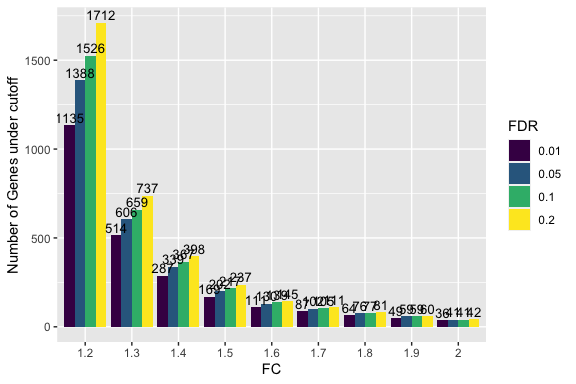Saving figures

Figures can be saved using two approaches:

1. Using imbedded fucntion with predetermined dpi

plot_cutoff(data = df,
plot.save.to = "cut_off_selection_plot.png")

2. Using ggsave from the ggplot2 library with option to customize the width, height and dpi.

library(ggplot2)
ggsave("cut_off_selection_plot.png", cutoff.result[], width = 5, height = 5, dpi = 300)

### 1.2 Cutoff Plot - Input: a list.

cutoff.result.list <- plot_cutoff(data = d1,
comp.names = c('a', 'b'))

The result object cutoff.result.list takes a list as an input data and contains 2 objects:

1. A table that summarizes the number of DE genes under threshold combination for each of the data frames in the list.

head(cutoff.result.list[])
Comparisons.ID pvalue FC Number_of_Genes
a 0.01 1.2 1135
a 0.05 1.2 1388
a 0.1 1.2 1526
a 0.2 1.2 1712
a 0.01 1.3 514
a 0.05 1.3 606

2. A plot to visualize it. A 3D plotly object is not created for a list input data.

cutoff.result.list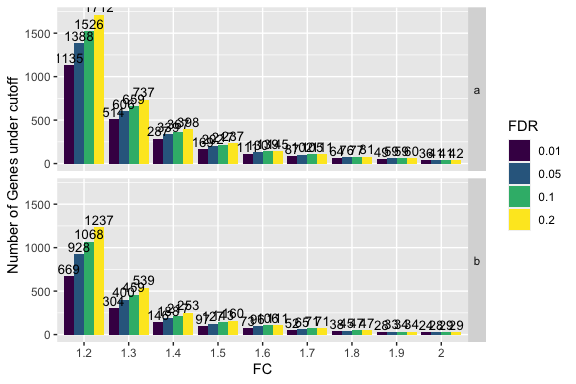Saving figures

Figures can be saved using two approaches:

1. Using imbedded fucntion with predetermined dpi

plot_cutoff(data = d1,
comp.names = c("A", "B"),
plot.save.to = "cut_off_list_plot.png")

2. Using ggsave from the ggplot2 library with option to customize the width, height and dpi.

library(ggplot2)
ggsave("cut_off_list_plot.png", cutoff.result.list, width = 5, height = 5, dpi = 300)

## QQ Plot

This is the function to generate a qqplot object with confidence interval from the input data. The input data is a summary statistics table or a list that contains multiple summary statistics tables from limma or DEseq2, where each row is a gene.

### 2.1 QQ Plot - Input: a data frame.

qq.result <- plot_qq(df)
qq.result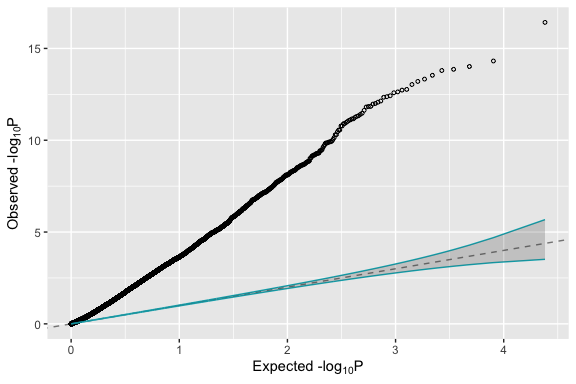Saving figures

Figures can be saved using two approaches:

1. Using imbedded fucntion with predetermined dpi

plot_qq(data = df,
plot.save.to = "qq_plot.png")

2. Using ggsave from the ggplot2 library with option to customize the width, height and dpi.

library(ggplot2)
ggsave("qq_plot.png", qq.result, width = 5, height = 5, dpi = 300)

### 2.2 QQ Plot - Input: a list.

plot_qq function can also take a list as an input data, but requires comp.names to be specified. The result object is a set of qq plots for each of the data frames in the list.

qq.list.result <- plot_qq(data = d1,
comp.names = c('A', 'B'))
qq.list.result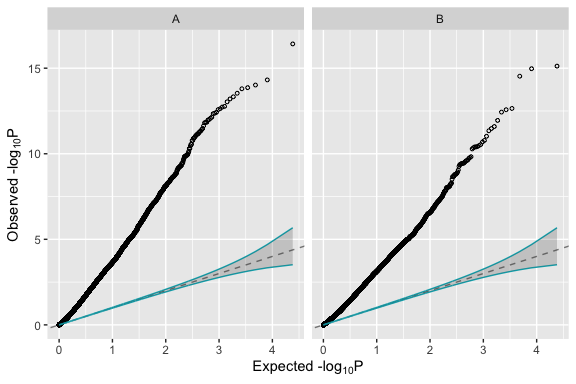Saving figures

Figures can be saved using two approaches:

1. Using imbedded fucntion with predetermined dpi

plot_qq(data = d1,
comp.names = c("A", "B"),
plot.save.to = "qq_list_plot.png")

2. Using ggsave from the ggplot2 library with option to customize the width, height and dpi.

library(ggplot2)
ggsave("qq_list_plot.png", qq.list.result, width = 5, height = 5, dpi = 300)

## Volcano Plot

This is the function to process the summary statistics table generated by differential expression analysis like limma or DESeq2 and generate the volcano plot with the option of highlighting the individual genes or gene set of interest (like disease-related genes from Disease vs Healthy comparison). The input data is a summary statistics table or a list that contains multiple summary statistics tables from limma or DEseq2, where each row is a gene.

Below are the default parameters for plot_volcano. You can change them to modify your output. Use help(plot_volcano) to learn more about the parameters.

plot_volcano(
data = data,
comp.names = NULL,
geneset = NULL,
geneset.FCflag = "logFC",
highlight.1 = NULL,
highlight.2 = NULL,
upcolor = "#FF0000",
downcolor = "#0000FF",
plot.save.to = NULL,
xlim = c(-4, 4),
ylim = c(0, 12),
FCflag = "logFC",
highlight.FC.cutoff = 1.5,
highlight.FDR.cutoff = 0.05,
title = "Volcano plot",
xlab = "log2 Fold Change",
ylab = "log10(FDR)"
)

### 3.1 Volcano Plot - Input: a data frame.

plot_volcano(data = df)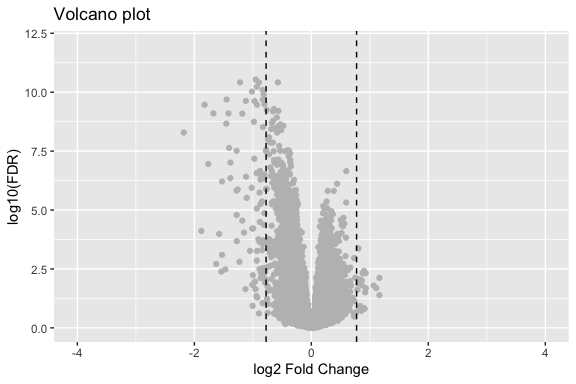### 3.2 Volcano Plot - Input: a list.

Volcano Plot can also take a list as an input data with specified comp.name for each data frame.

plot_volcano(data = d1,
comp.names = c('a', 'b'))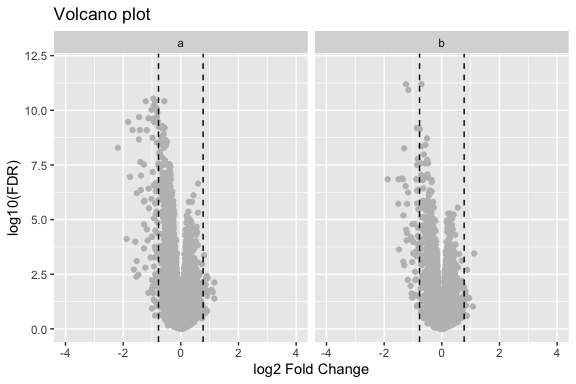### 3.3 Highlight genes of interest in the volcano plot

You can highlight gene sets (like disease related genes from a Disease vs Healthy comparison).

The gene set to be highlighted in the volcano plot can be spesified in two ways:

1. A summary statistics table with the highlighted genes as row names (the gene name format needs to be consistent with the main summary statistics table). For example, this summary statistics table could be the statistical analysis output from a Disease vs Healthy comparison (only containing the subsetted significant genes).

2. One or two vectors consisting of gene names. The gene name format needs to be consistent with the main summary statistics table. It can be set by the parameters highlight.1 and highlight.2. For example, you can assign the up-regulated gene list from the Disease vs Healthy comparison to highlight.1 and down-regulated gene list from the comparison to highlight.2.

Example using option 1 (use summary statistics table’s row name to highlight genes):

#disease gene set used to color volcanoplot
dgs <- RVA::Sample_disease_gene_set 

head(dgs)
logFC AveExpr t P.Value adj.P.Val B
ENSG00000176749.9 0.1061454 6.034635 -1.1704309 0.2446236 0.9188735 -5.6468338
ENSG00000086619.13 0.0862010 2.100165 -0.3331558 0.7397177 0.9989991 -5.7218518
ENSG00000198324.13 -0.1321791 5.702730 1.2768794 0.2046170 0.8889442 -5.5265238
ENSG00000134531.10 -0.4778738 4.562272 3.6721593 0.0003892 0.0298096 -0.1135281
ENSG00000116260.17 0.1842322 2.905702 1.6108394 0.1103830 0.7809130 -4.7560495
ENSG00000104518.11 0.1452149 -3.776628 0.2584838 0.7965675 0.9989991 -4.9101962

You can also specify the range of the plot by xlim and ylim.

plot_volcano(data = df,
geneset = dgs,
upcolor = "#FF0000",
downcolor = "#0000FF",
xlim = c(-3,3),
ylim = c(0,14))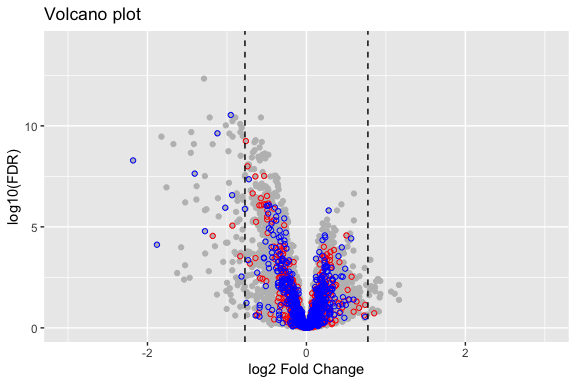By default, the genes which have positive fold change in the provided geneset parameter will be colored yellow, and negative fold will be colored purple, this also can be changed by specifying upcolor and downcolor:

plot_volcano(data = d1,
comp.names = c('a', 'b'),
geneset = dgs,
upcolor = "#FF0000",
downcolor = "#0000FF",
xlim = c(-3,3),
ylim = c(0,14))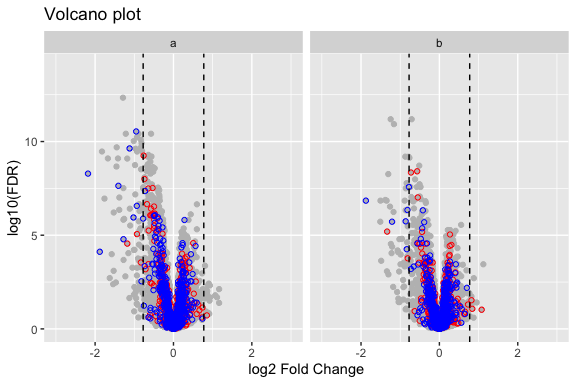Example with option 2 You can also specify the color of highlight.1 with upcolor parameter and highlight.2 with downcolor parameter.

volcano.result <- plot_volcano(data = df,
highlight.1 = c("ENSG00000169031.19","ENSG00000197385.5","ENSG00000111291.8"),
highlight.2 = c("ENSG00000123610.5","ENSG00000120217.14", "ENSG00000138646.9", "ENSG00000119922.10","ENSG00000185745.10"),
upcolor = "darkred",
downcolor = "darkblue",
xlim = c(-3,3),
ylim = c(0,14))
volcano.result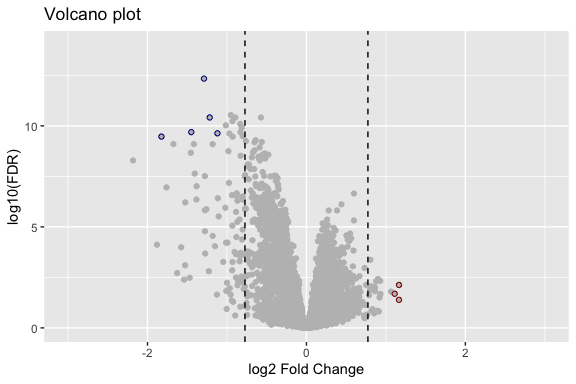Saving figures

Figures can be saved using two approaches:

1. Using imbedded fucntion with predetermined dpi

plot_volcano(data = df,
geneset = dgs,
plot.save.to = "volcano_plot.png")

2. Using ggsave from the ggplot2 library with option to customize the width, height and dpi.

library(ggplot2)
ggsave("volcano_plot.png", volcano.result, width = 5, height = 5, dpi = 300)

## Pathway analysis plot

This is the function to do pathway enrichment analysis (and visualization) with rWikiPathways (also KEGG, REACTOME & Hallmark) from a summary statistics table generated by differential expression analysis like limma or DESeq2. In directional enrichment analysis, the sign(positive/negative) indicates the direction of the enrichement, not the mathematical sign of -log10(FDR), for example, the positive sign indicate the up-regulated genes in geneset is over-represented in all the genes in the genome are up-regulated.

Below are the default parameters for plot_pathway. You can change them to modify your output. Use help(plot_pathway) to learn more about the parameters.

plot_pathway(
data = df,
comp.names = NULL,
gene.id.type = "ENSEMBL",
FC.cutoff = 1.3,
FDR.cutoff = 0.05,
FCflag = "logFC",
Fisher.cutoff = 0.1,
Fisher.up.cutoff = 0.1,
Fisher.down.cutoff = 0.1,
plot.save.to = NULL,
pathway.db = "rWikiPathways"
)

Our sample dataset provided in the package only contains ENSEMBL gene id types. Other types can be used by changing the parameter gene.id.type = " id type". When inputing a single data frame for analysis, comp.names are not required. Currently we are using rWikiPathways as a database for enrichment analysis but this can be changed to KEGG, REACTOME, Hallmark or a static version of rWikiPathways by changing the parameter pathway.db = "database name".

pathway.result <- plot_pathway(data = df, pathway.db = "Hallmark", gene.id.type = "ENSEMBL")

### 4.1 Pathway analysis result is a list that contains 5 objects:

1. Pathway analysis table with directional result (test up-regulated gene set and down-regulated gene set respectively).

head(pathway.result[])
HALLMARK_INTERFERON_ALPHA_RESPONSE HALLMARK_INTERFERON_ALPHA_RESPONSE 0e+00 down -43.896635 #1F78B4
HALLMARK_INTERFERON_GAMMA_RESPONSE HALLMARK_INTERFERON_GAMMA_RESPONSE 0e+00 down -48.427295 #1F78B4
HALLMARK_TNFA_SIGNALING_VIA_NFKB HALLMARK_TNFA_SIGNALING_VIA_NFKB 0e+00 down -18.950395 #1F78B4
HALLMARK_INFLAMMATORY_RESPONSE HALLMARK_INFLAMMATORY_RESPONSE 0e+00 down -15.856884 #1F78B4
HALLMARK_IL6_JAK_STAT3_SIGNALING HALLMARK_IL6_JAK_STAT3_SIGNALING 0e+00 down -8.161561 #1F78B4
HALLMARK_COMPLEMENT HALLMARK_COMPLEMENT -4e-07 down -6.425707 #1F78B4

2. Pathway analysis table with non-directional fisher’s enrichment test result for all DE genes regardless of direction.

head(pathway.result[])

3. Pathway analysis plot with directional result.

pathway.result[]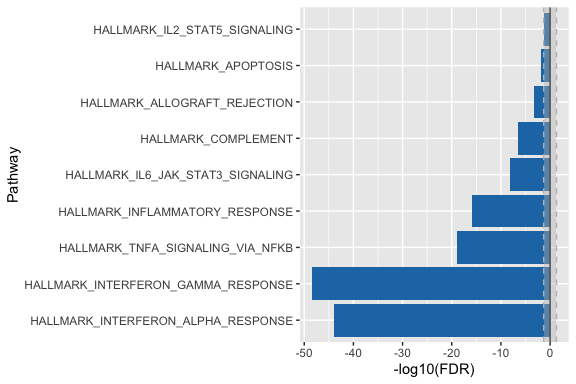4. Pathway analysis plot with non-directional result.

pathway.result[]
NULL

5. Pathway analysis plot with combined direaction and non-directional result.

pathway.result[]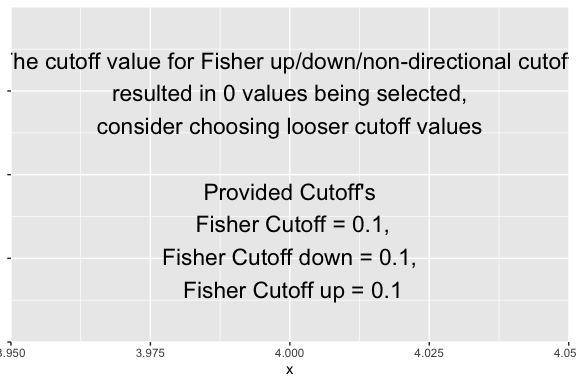Saving figures

Figures can be saved using ggsave from the ggplot2 library.

library(ggplot2)
ggsave("joint_plot.png",pathway.result[], width = 5, height = 5, dpi = 300)

### 4.2 Pathway analysis for the list of summary tables will result in a list that contains 4 objects:

Pathways with list of data as input, the list can be replaced with d1 from the top. When list inputs are given comp.names should be speicified in order to identify the comparison groups.

list.pathway.result <- plot_pathway(data = list(df,df1),comp.names=c("A","B"),pathway.db = "Hallmark", gene.id.type = "ENSEMBL")

1. Pathway analysis table with directional result for all datasets submited.

head(list.pathway.result[])
A HALLMARK_INTERFERON_ALPHA_RESPONSE HALLMARK_INTERFERON_ALPHA_RESPONSE 0e+00 down -43.896635 #1F78B4
A HALLMARK_INTERFERON_GAMMA_RESPONSE HALLMARK_INTERFERON_GAMMA_RESPONSE 0e+00 down -48.427295 #1F78B4
A HALLMARK_TNFA_SIGNALING_VIA_NFKB HALLMARK_TNFA_SIGNALING_VIA_NFKB 0e+00 down -18.950395 #1F78B4
A HALLMARK_INFLAMMATORY_RESPONSE HALLMARK_INFLAMMATORY_RESPONSE 0e+00 down -15.856884 #1F78B4
A HALLMARK_IL6_JAK_STAT3_SIGNALING HALLMARK_IL6_JAK_STAT3_SIGNALING 0e+00 down -8.161561 #1F78B4
A HALLMARK_COMPLEMENT HALLMARK_COMPLEMENT -4e-07 down -6.425707 #1F78B4

2. Pathway analysis table with non directional result for all datasets submited.

head(list.pathway.result[])
HALLMARK_KRAS_SIGNALING_DN…1 A HALLMARK_KRAS_SIGNALING_DN HALLMARK_KRAS_SIGNALING_DN 0.0167355 0.4983117
HALLMARK_APICAL_SURFACE…2 A HALLMARK_APICAL_SURFACE HALLMARK_APICAL_SURFACE 0.1609079 0.6585765
HALLMARK_KRAS_SIGNALING_DN…3 B HALLMARK_KRAS_SIGNALING_DN HALLMARK_KRAS_SIGNALING_DN 0.0029075 0.0930402
HALLMARK_APICAL_SURFACE…4 B HALLMARK_APICAL_SURFACE HALLMARK_APICAL_SURFACE 0.0040452 0.0930402

3. Pathway analysis plot with directional result for list of summary tables.

list.pathway.result[]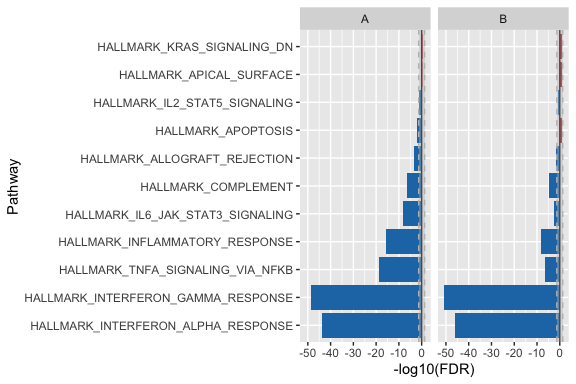4. Pathway analysis plot with non directional result for list of summary tables.

list.pathway.result[]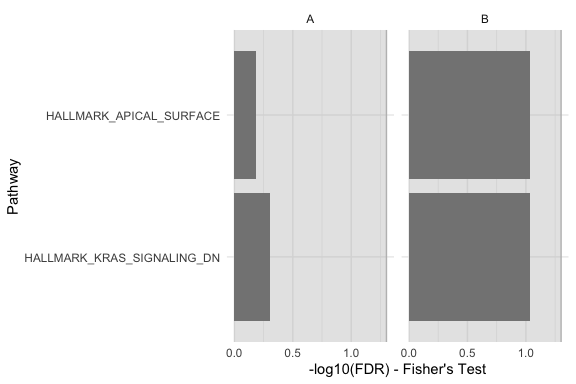Saving figures

Figures can be saved using ggsave from the ggplot2 library.

library(ggplot2)
ggsave("non-directional.png",pathway.result[], width = 5, height = 5, dpi = 300)

## Heatmap

### 5.1 Heatmap

You can plot a heatmap from raw data rather than a summary statistics table. plot_heatmap.expr has the ability to calculate average expression values and change from baseline. Importantly, these calculations do not calculate statistical signifance or correct for confounding factors - they should not be used as statistical analyses but as data overviews.

For this, you need a count table and annotation table. The count table should have the geneid as row names and the samples as column names. These column names must match the sample.id column in your annotation file:

count <- RVA::count_table[,1:50]

count[1:6,1:5]
A1 A10 A11 A12 A13
ENSG00000121410.11 2 5 4 2 2
ENSG00000166535.19 0 0 0 0 0
ENSG00000094914.12 405 493 422 346 260
ENSG00000188984.11 0 0 0 0 0
ENSG00000204518.2 0 0 0 0 0
ENSG00000090861.15 555 782 674 435 268

annot <- RVA::sample_annotation[1:50,]

head(annot)
sample_id tissue subject_id day Treatment subtissue
A1 Blood 1091 0 Treatment_1 Blood
A10 Blood 1095 14 Placebo Blood
A11 Blood 1095 28 Placebo Blood
A12 Blood 1097 0 Placebo Blood
A13 Blood 1097 14 Placebo Blood
A14 Blood 1097 28 Placebo Blood

Plot a simple summary of expression values:

Use help(plot_heatmap.expr) for more information on the parameters.

hm.expr <- plot_heatmap.expr(data = count,
annot = annot,
sample.id = "sample_id",
annot.flags = c("day", "Treatment"),
ct.table.id.type = "ENSEMBL",
gene.id.type = "SYMBOL",
gene.names = NULL,
gene.count = 10,
title = "RVA Heatmap",
fill = "CPM",
baseline.flag = "day",
baseline.val = "0",
plot.save.to = NULL,
input.type = "count")

The result of plot_heatmap.expr with fill = CPM contains 2 objects:

1. Heat map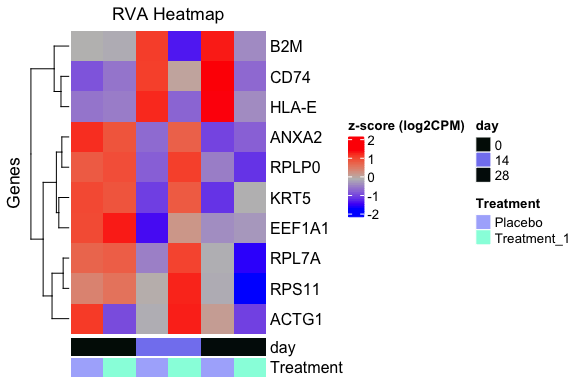2. A data frame of CPM values (fill = CPM in this example) for each geneid split by treatment group and time point.

head(hm.expr[])
geneid 0_Placebo 0_Treatment_1 14_Placebo 14_Treatment_1 28_Placebo 28_Treatment_1
ENSG00000019582 12.88519 12.95117 13.35188 13.10461 13.44575 12.92982
ENSG00000089157 13.53517 13.56759 13.09506 13.60246 13.16606 12.99473
ENSG00000142534 12.74876 12.79580 12.62589 12.97305 12.60354 12.21103
ENSG00000148303 12.96778 12.98249 12.68783 13.03604 12.77388 12.45019
ENSG00000156508 15.57407 15.63724 15.13047 15.42868 15.31821 15.33419
ENSG00000166710 13.69652 13.68064 14.08861 13.28912 14.16103 13.59287

Customize the plot & Save the figure

Here is an example of how you can customize your output dimensions and save your new output using the png() function. Always make sure that the ComplexHeatmap library is loaded for the draw function.

library(ComplexHeatmap)
png("heatmap_plots2cp.png", width = 500, height = 500)
draw(hm.expr$gp) dev.off() To calculate CFB from your input data, you must specify the baseline. The heatmap shown below compares each treatment on days 14 and 28 to the respective treatment on day 0. Use help(plot_heatmap.expr) for more information on the parameters. hm.expr.cfb <- plot_heatmap.expr(data = count, annot = annot, sample.id = "sample_id", annot.flags = c("day", "Treatment"), ct.table.id.type = "ENSEMBL", gene.id.type = "SYMBOL", gene.names = NULL, gene.count = 10, title = "RVA Heatmap", fill = "CFB", baseline.flag = "day", baseline.val = "0", plot.save.to = NULL, input.type = "count") The result of plot_heatmap.expr with fill = CFB contains 2 objects: 1. Heat map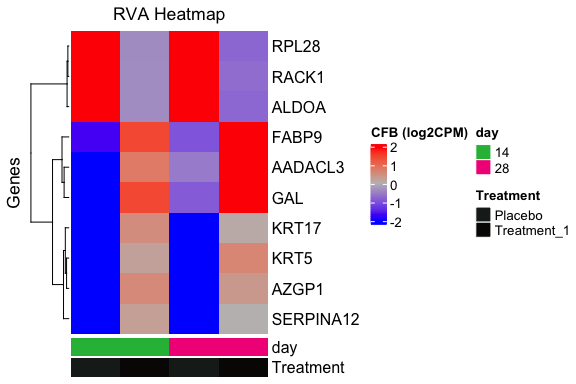2. A data frame of change from baselines values (fill = CFB in this example) for each geneid split by treatment group and time point. head(hm.expr.cfb[]) geneid 14_Placebo 14_Treatment_1 28_Placebo 28_Treatment_1 ENSG00000069482 -3.120986 1.4161927 -0.9142305 2.3416320 ENSG00000108107 2.930098 -0.3906393 2.9803268 -0.7834090 ENSG00000128422 -2.060845 0.5122622 -2.6034678 0.0996217 ENSG00000149925 2.735214 -0.3762279 2.6791153 -0.7753080 ENSG00000160862 -2.688269 0.5648108 -2.5291108 0.3503731 ENSG00000165953 -3.160729 0.2600754 -2.5078464 0.0210713 Customize the plot & Save the figure Here is an example of how you can customize your output dimensions. library(ComplexHeatmap) png("heatmap_plots1cf.png", width = 500, height = 500) draw(hm.expr.cfb$gp)
dev.off()

## Gene expression

### 6.1 Gene expression

Let’s load in the sample data provided in this package. Note that the count table containing data must have the geneid set as the rownames and must have column names which match the sample.id column of the annotation file.

anno <- RVA::sample_annotation

head(anno)
sample_id tissue subject_id day Treatment subtissue
A1 Blood 1091 0 Treatment_1 Blood
A10 Blood 1095 14 Placebo Blood
A11 Blood 1095 28 Placebo Blood
A12 Blood 1097 0 Placebo Blood
A13 Blood 1097 14 Placebo Blood
A14 Blood 1097 28 Placebo Blood

ct <- RVA::sample_count_cpm

ct[1:6,1:5]
A1 A10 A11 A12 A13
ENSG00000166535.19 8.5629 7.6227 7.7743 7.6845 8.5539
ENSG00000094914.12 3.1405 8.2261 7.9616 8.1047 7.8747
ENSG00000188984.11 5.7477 7.7889 8.0268 7.8954 8.0294
ENSG00000090861.15 8.2753 8.1688 8.6159 7.3708 7.7271
NA NA NA NA NA NA
NA.1 NA NA NA NA NA

Below is a simple plot using the defaults. Further parameter changes can allow you to change the log scaling, the input type to either cpm or count, and the genes selected for plotting. The sample table we are using already has data points as CPM, so we will use CPM as our input.type.

Use help(plot_gene) for more information on the parameters.

gene.result <- plot_gene(ct,
anno,
gene.names = c("AAAS", "A2ML1", "AADACL3", "AARS"),
ct.table.id.type = "ENSEMBL",
gene.id.type = "SYMBOL",
treatment = "Treatment",
sample.id = "sample_id",
time = "day",
log.option = TRUE,
plot.save.to = NULL,
input.type = "cpm")

The result of plot_gene contains 2 objects:

1. A gene expression plot that distinguishes log cpm gene expression for each geneid across the treatment groups and time points.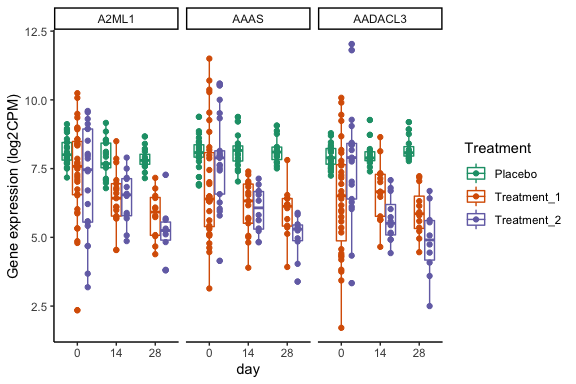2. A table that shows gene expression values by gene id, treatment group and time point with both sample ids and gene symbols.

head(gene.result[])
geneid sample_id exprs Treatment day SYMBOL
ENSG00000166535 A1 8.5629 Treatment_1 0 A2ML1
ENSG00000166535 A10 7.6227 Placebo 14 A2ML1
ENSG00000166535 A11 7.7743 Placebo 28 A2ML1
ENSG00000166535 A12 7.6845 Placebo 0 A2ML1
ENSG00000166535 A13 8.5539 Placebo 14 A2ML1
ENSG00000166535 A14 7.9185 Placebo 28 A2ML1

Customize the plot & Save the figure

Here is an example of how you can customize your output dimensions and save your new plot using the ggsave function. Always make sure that the ggplot2 library is loaded.

library(ggplot2)
ggsave(gene.result, "gene_plots1_4.png", device = "png", width = 100, height = 100, dpi = 200, limitsize = FALSE)